Geometrical determinations of IMRT photon pencil-beam path in radiotherapy wedges and limit divergence angle with the Anisotropic Analytic Algorithm (AAA)

Geometrical determinations of IMRT photon pencil-beam path in radiotherapy wedges and limit divergence angle with the Anisotropic Analytic Algorithm (AAA)

Society for Industrial and Applied Mathematics (SIAM), Denver, Colorado, USA.

Cite this article as: Casesnoves F. Geometrical determinations of IMRT photon pencil-beam path in radiotherapy wedges and limit divergence angle with the Anisotropic Analytic Algorithm (AAA). Int J Cancer Ther Oncol 2014; 2(3):02031.
DOI:
http://dx.doi.org/10.14319/ijcto.0203.1

Original Article

Abstract

Purpose: Static wedge filters (WF) are commonly used in radiation therapy, forward and/or inverse planning. We calculated the exact 2D/3D geometrical pathway of the photon-beam through the usual alloy WF, in order to get a better dose related to the beam intensity attenuation factor(s), after the beam has passed through the WF. The objective was to provide general formulation into the Anisotropic Analytical Algorithm (AAA) model coordinates system (depending on collimator/wedge angles) that also can be applied to other models. Additionally, second purpose of this study was to develop integral formulation for 3D wedge exponential factor with statistical approximations, with introduction for the limit angle/conformal wedge.

Methods: The radiotherapy model used to develop this mathematical task is the classical superposition-convolution algorithm, AAA (developed by Ulmer and Harder). We worked with optimal geometrical approximations to make the computational IMRT calculations quicker/reduce the planning-system time. Analytic geometry/computational-techniques to carry out simulations (for standard wedges) are detailed/developed sharply. Integral developments/integral-statistical approximations are explained. Beam-divergence limit Angle for optimal wedge filtration formulas is calculated/sketched, with geometrical approximations. Fundamental trigonometry is used for this purpose.

Results: Extent simulation tables for WF of 15º, 30º, 45º, and 60º are shown with errors. As a result, it is possible to determine the best individual treatment dose distribution for each patient. We presented these basic simulations/numerical examples for standard manufacturing WF of straight sloping surface, to check the accuracy/errors of the calculations. Simulations results give low RMS/Relative Error values (formulated) for WF of 15º, 30º, 45º, and 60º.

Conclusion: We obtained a series of formulas of analytic geometry for WF that can be applied for any particular dose delivery model. Simulations results gave acceptable trigonometrical approximations/data that can be used for LINAC applications/planning-system software. The integral formulas presented are practical for dose delivery calculations/3D-approximations when using WF/other similar types of beam modification devices. Limit angle formulation and conformal wedge concept was also presented.

Keywords: Radiotherapy; Intensity Modulated Radiation Therapy; Static Alloy Wedges; Analytic Geometry; Treatment Planning Optimization (TPO); Analytic Anisotropic Algorithm (AAA); Superposition-Convolution Models (SCM)

Introduction

Currently, radiotherapy treatment is based on two main objectives: (1) First, to deliver the optimal radiation at Planning Target Volume(s) (PTVs), and (2) secondly, to minimize the dose magnitude in the Organs at Risk (OARs), and surrounding PTV tissues. Therefore, the radiation delivery should conform the tumor shape with the most convenient approximation(s).

There are a number of reasons to consider conformal and optimized radiotherapy as the most important objective for cancer treatment when radiation is included into the general treatment planning of the disease. The optimal dose guarantees a standard level of life quality, and maximum statistical survival time for the patient. Survival time has recently been determined, with clinical trials, when the tumor treatment is a combination of methods (RT and Chemotherapy, usually). This fact implies that when the optimal dose distribution is reached, the most desirable pharmacological effect will appear in the PTV (direct) and OARs (inverse). The explanation is that PTVs tumor cells will be killed or seriously damaged by radiation prior to the drug's action, and just the inverse process occurs at OARs regions (that is, the less radiation on OARs, the better natural cell conditions to avoid/minimize side-effects of drugs).

Nevertheless, apart from the principal mechanical and physical tools and parts of the new accelerators, the complementary use of Beam Modification Devices (BMDs) constitute an effective and easy way to get conformal dosage, without excessive technical effort, and waste of treatment time. The inconvenient of the BMDs is the dose distribution alteration, due mainly to the increment of scattering photons (more frequent in Kilovoltage than Megavoltage). BMD types are classified by Shielding, Compensation, Wedge Filtration and Flattering. There are a number of BMDs, among them, those most frequently used are Multileaf Collimators (MLC), Shielding, Compensators, Wedge Filters, and Penumbra Trimmers. We focus this paper on Wedge Filters, which are used in general for relatively superficial tumors. Wedges can be classified as: Universal, Dynamic, Virtual, and Pseudo-Wedges.7

The use of wedges is justified for practical, technical and economic reasons. High-quality alloy materials are not expensive, and the size of the wedges makes their handling, change and substitution easy. Manufacturing of wedge filter series with different angles (usually 15º, 30º, 45º, and 60º) is neither difficult nor expensive. Alloy is a high-endurance material which provides long industrial life for continuous RT sessions. In addition, several combinations of wedges in 2D and 3D configurations may be used to obtain a series of radiation distribution(s) to adapt the dose delivery to the tumor shape with accurate approximations.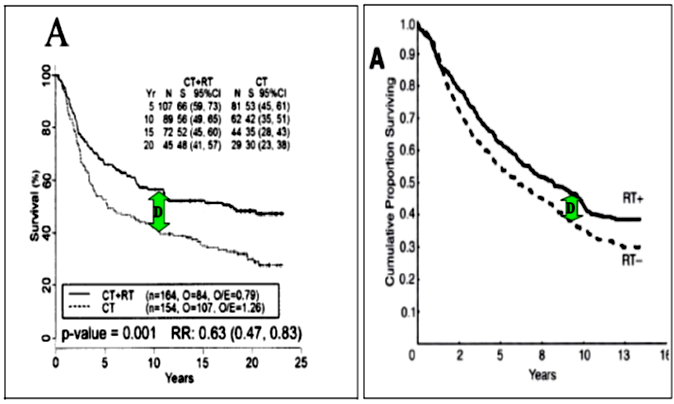FIG. 1:
Two significant trial-statistical results about survival time for Breast (left) and Rectal cancer (right) [Cancer Study of Ragaz & Colleagues.2 The green arrows show the difference between the alternative treatments for both tumors. For Breast Cancer, the upper curve corresponds to the survival time with RT-Chemo, and the lower to only Chemotherapy. For Rectum tumors, the upper curve is for RT with Surgery, and the lower is for only Surgery. The difference of survival time is high from the approximately the 2nd - 3rd year after diagnosis-treatment on. In breast cancer the difference of survival reaches, in some cases, a ratio higher than 20%.

However, some of the approximations published do not take into account the specific 3D geometry of the wedge filter, and avoid developing a formal general-geometrical calculation based on a 3D planes intersection at an alpha angle (the proper wedge angle). Therefore, both exact and approximated calculations may be useful to add accuracy and simplicity to the planning software, through practical formulas. What is more, the wedge manufacturing can be designed and adapted to the specific conditions of each accelerator type. And also the materials could be improved to carry out a precise and clean attenuation and filtration of the beam, avoiding big dispersion, (contamination particles, mainly electrons, forward/backward scatter, and others).

It is useful and efficacious to generate knowledge about the different wedge options and combinations available to complement the treatment protocols; RT in general, External Beam Radiotherapy (EBRT), and IMRT. The direct practical result is a series of wedge options to be selected by planners according to the specific tumor types and shapes. The Coordinate System is a practical reference in TPO which can be used as general starting point to determine dose distribution and obtain equations to develop the coordinate/planning system. These geometrical formulas can be adapted or modified in IMTR for the different technical and mechanical options.

Therefore, the aim of this technical paper is to provide simple geometrical calculations and approximations for wedge use. We have developed the 2D/3D geometrical photon pencil-beam path through the wedge. The selected model is the AAA algorithm which integrates an attenuation factor into the integral formula, when wedges are used for conformal dose distribution. This exponential attenuation factor depends on the 3D pathway of the pencil beam through the wedge and its material. Secondarily, some approximations are derived from the principal exact formulas, whose objective is to provide practical mathematics for the planning system software (e.g., to avoid sine, cosine, complicated quadratic expressions, etc.). Limit Divergence Angle (LA) for optimal positioning of beam is defined, formulated, and sketched.

In brief, the objective of this contribution is practical and technical, in such a way that any RT researcher could develop and improve further formulation for other RT Models/Dose Delivery Systems. Finally, Simple Simulations are developed to determine error magnitudes, with complementary information about the mathematical development. Results obtained in a simulation series, e.g., for 30º wedges, show acceptable RMS errors (~10-2) for the approximations carried out. Simulations are based on random values, and located at the positive quadrant (broad wedge part) of the wedge coordinate system.

Methods and Materials

The Anisotropic Analytic Algorithm (AAA)
The Analytic Anisotropic Algorithm, AAA, is a well-known and extensively used Superposition-Convolution Model in RT. AAA is evolved from an initial Integral Superposition Convolution Model, whose parameters were optimized using large Monte Carlo experimental data in water. The starting Physical Equation to develop the model 8-11 was a Yukawa Kernel based on the formulation structure of the classical Yukawa Gaussian Potential for Electromagnetism, as follows,in the transverse plane at depth z. The characteristic function I(z) denotes the area integral of the dose over the transverse plane of the pencil beam at depth z, normalized to one photon, and σ2k(Z) is the mean square radial displacement of the profile at depth z. Next, a mathematical development based also in experimental data and Fourier Transform, was carried out 8-11, to transform the initial formula on a triple sum of Gaussians (Superposition) from the initial simple Gaussian, and optimize the coefficients according to photon beam experimental data. As a result, the Pencil Model Dose at a depth z and into an almost differential cylinder (Triple Gaussian Pencil Beam) whose diameter is 2r is,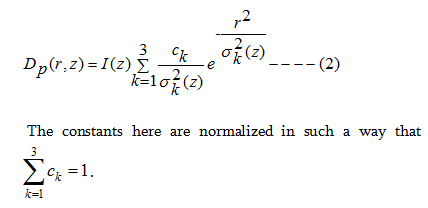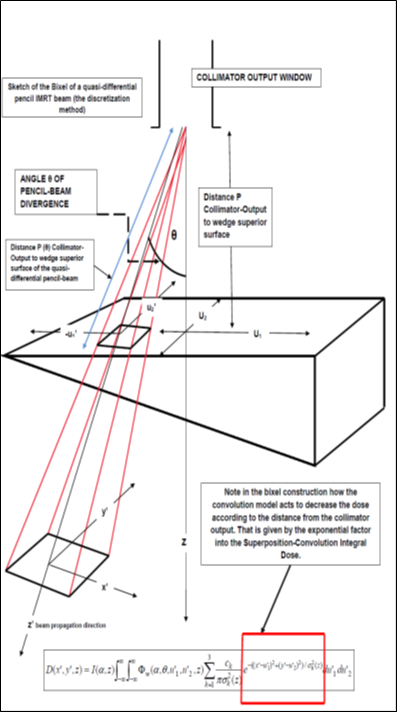FIG. 2: Sketch of a bixel (a quasi-differential rectangular prism (a pyramid), whose apex is at collimator output) which contains a Pencil-beam whose path goes through the wedge with a divergence angle θ. A Voxel is a quasi-differential portion of volume almost parallelepipedic). We detail complementary explanations about the Superposition-Convolution method to introduce the mathematical concept. Erratum: the limits of the Convolution Integral for the bixel are not infinity and minus infinity. The limits are the values of u1' and u2' for the pixel of the wedge surface that correspond to the selected bixel. To carry out calculations for a complete Treatment Planning Optimization and Computational Software Development, it is necessary to also include the rotation angles of the gantry and the couch. This done by implementing the corresponding matrices of rotations, and taking into account the Isocentre Position. This matter goes beyond the scope of this Technical Paper, which is focused only on the wedges Pencil-Beam pathway. Note also that this Superposition-Convolution Integral Model corresponds to the initial stages of the AAA algorithm development in water (constant density). It is not too complicated to implement these calculations on the recent AAA Formulas fitted for heterogeneus tissues with the necessary correction factors.Erratum:in convolution formula z must be z'.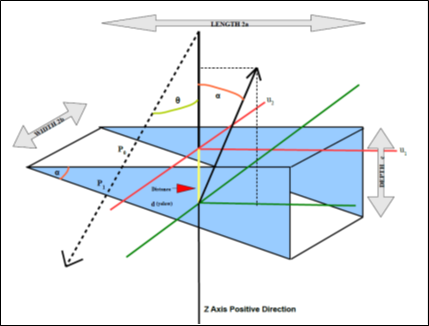FIG. 3: The intent here is to provide an easy path to understanding of the Geometrical method. The unitary vector n, which is perpendicular to the inferior plane, is sketched with its projections. An arbitrary Pencil-Beam is drawn on the left to show the input and output points upon the wedge volume. The broad part of the wedge corresponds to the positive quadrant of the superior U plane. Simulations were carried out just in this part, at the positive quadrant.

Note that the positive Z-axis is towards the Isocenter and patient surface. In the articles of foundation of the AAA Model, the measurements of the wedge are taken similar to the pictured one. That is, 2a, 2b, and 2c (we choose c instead 2c for simplicity).

We remind that a vector in 3D Analytic Geometry is defined by the difference of two points. The directional point (arrow) and the origin point (usually at coordinate center). In the calculations the origin of the perpendicular vector is taken at coordinate centre, but in the picture is translated at the inferior plane surface to put forward the problem geometry with clarity.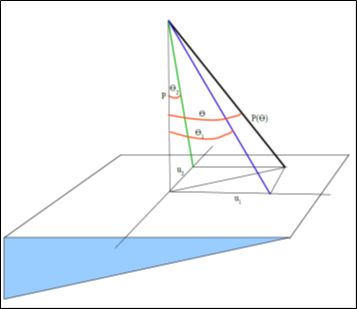FIG. 4: We show the Pencil-Beam Divergence Angle subdivision to get smaller angles in order to better approximate the tangent value by Taylor Series, to the angle value in radians (first term of series, more options with more terms could be useful, but these are not linear). Note that this positive quadrant is put at the broad part of the wedge for simulations.

All the parameters are tabulated.8-11 The derivation of the coefficient ck with the help of the Fourier transform, have already been described in the papers.8-9 Tabulations of I(z), σk(z), and ck, based on Monte Carlo calculations of photon pencil beams for Co-60 gamma radiation and bremsstrahlung from 6 to 18 MV, have already been published.8-9 This triple-Gaussian representation of the pencil beam has been chosen because its convolution with the photon flux distribution Ф(x, y, z) at depth z can be analytically performed in many practical cases. An important contribution to the saving of computer time and storage space is thereby achieved, because numerical convolutions or applications of look-up tables from their fitting formulas are partially avoided. The analytical form of the resulting dose distributions may also offer other, yet unknown, applications. The triple-Gaussian pencil beam approach can be applied to radiation beam profiles that represent rectangular satellite blocks and wedge filters, as it is the case of this paper. The derivation of the coefficients ck with the help of the Fourier transform, have already been described in the papers.9-11 The term 'Superposition' comes from the sum of three Gaussians into the integral. The term 'Convolution' comes from the mathematical transformation carried out into the Dose-Deposition Kernel at the Integral.

With this Triple Gaussian Dp (r, z), a Kernel K (x, y, u, v) was constructed to implement the dose term into the integral expression for the initial Superposition-Convolution Model in water, and then, the integral dose results in general as follows,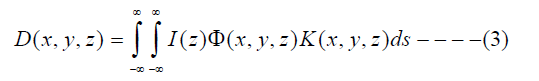where I(z) is the area integral of the absorbed dose over a plane perpendicular to the pencil beam axis at depth z per incident photon , Φ is the photon flux distribution of the beam per unit of intensity, and K is the kernel expression corresponding to the PBM, which is called the PB dose kernel, and describes the spatial distribution of the absorbed energy. This kernel could perfectly include any other PBM, for example the classic Anesjö model 12 or others. Now we focus on the aim of this research. The complete Triple Gaussian Pencil beam Model for water, when using wedges of angle α and taking into account the Collimator Divergence Angle (usually very small), θ then reads (see Figure 2),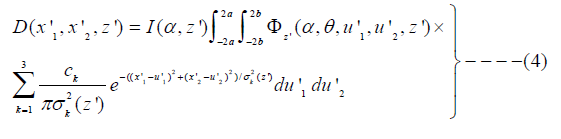Eq (4) [erratum, limits of integral -a, a, -b, b]

The Flux (and the Intensity) change with an exponential attenuation factor that depends on the alloy material, μw, and the pathlength through the wedge LK in this way,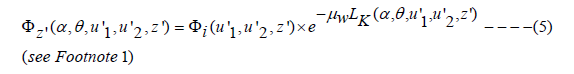This point will be explained in detail in the next subsection. The intention here is to show the complete formula used to obtain the geometrical results, as we are only interested in the determination of LK. Recently 9, a new contribution by the same authors for a 3D superposition-convolution algorithm based on their already developed, Triple Gaussian Pencil Beam Model (TGPBM). This algorithm has been built up from results of Monte Carlo calculations. This superposition-convolution algorithm sorts the density changes of the tissue heterogeneities. An essential result of this algorithm is the determination of build-up and build-down effects in the domain of the density discontinuities. The corresponding practical measurements show a standard deviation of about 2%. We won´t describe the long theoretical framework and development of this algorithm, because what is of practical importance for the understanding is that the complete dose distribution formula (41) of the article 10-11 for heterogeneous tissues reads,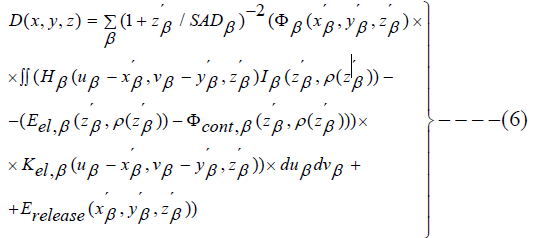Where β is the number of pencil beams, SADβ is the focus to surface distance of the pencil β, Φβ is the flux for the β pencil beam, Hβ is the kernel containing the pencil beam model (we can choose the model (that is, H (u-x´,v-y´,z´)). Iβ is the area integral of the absorbed dose and its expression is given in the formula (33) of the Ulmer et al articles 8-11, Eel,β is the energy of the electrons in the β pencil beam given again by formula (22), Φel,cont is the flux of the contamination particles, mainly electrons, and given by formula (33), Kel,β is the dose deposition kernel due to contamination electrons and is adapted by formula (23). Finally, Erelease is the energy released by local disequilibrium of forward and backward scatter and given by the formula (38). To implement in software and use this formula in mathematical calculations may appear to be rather difficult, but several parts have a simple development and others result null in specific cases.Where Φz' (α, θ, u’1, u’2, z) is of the form (depending on the wedge angle alpha and the divergence angle theta):Because of we apply the Inverse Square Law (ISL) of flux propagation for photons. (We detail the mathematical development of the ISL applied on Flux calculations;  we have at collimation output point a flux,  Ф0 , then, at wedge surface (still air) we get  (ISL) as detailed in [Eq 9], then and at any point z’ of the bixel, for the same ISL, which is the same expression of [Eqs 8, 9].) That is, we take as reference the wedge surface flux for ФW and this value decreases along the z coordinate of the pencil beam according to the ISL. In the same way, the variation of the coordinates values from the pixel of wedge filter surface to the bixel base can be determined by trigonometric proportions from the triangles of the quasi-differential pyramid of the bixel as follows,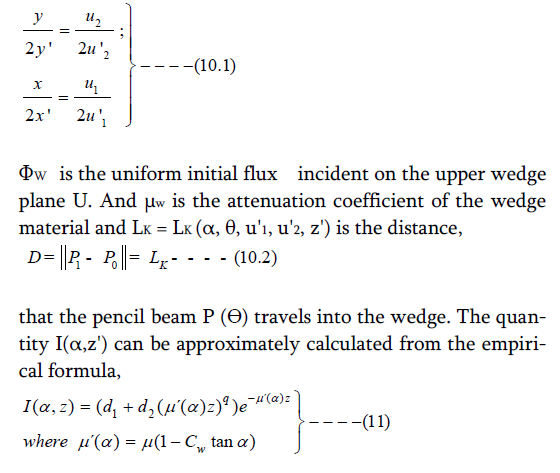However, the calculation of Intensity related to wedge angle is not the focus of the paper. These formulas are well detailed in the references about the primary stages of the AAA model. The parameters d1, d2, μ, q, and Cw are tabulated.9,10

The modification is required because the wedge changes the energy spectrum of the photon beam. The integral detailed above is only an approximation for the dose, since the patch of the patient surface is not necessarily a plane, which is oriented transversally to Z' axis.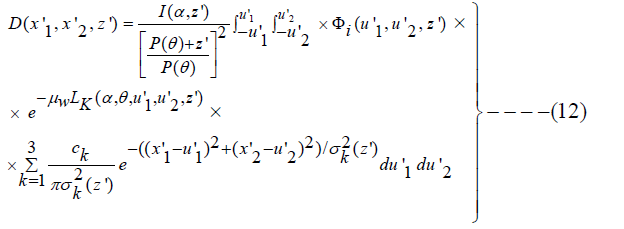In this Formula we have set the limits for the Coordinates u'1 and u'2 related to the difference of vectors u1 and u2 that define the Coordinates System of the Central Axis Beam. There are other techniques to sort these calculation(s). Here LK (α, θ, u'1, u'2, z') is the distance D of the pathway through the wedge. This integral is developed numerically (Gauss-Quadrature preferably) to obtain the dose for this bixel. To determine the total dose-deposition, it is necessary to add another summation which gathers all the bixels which comprise totally the wedge surface, and therefore the summation of the Pencil-Beams (each one with proper characteristics as it is done in IMRT).13-16

Geometrical calculations

In this Section we show the Analytic Geometry calculations to obtain the exact length of the pathway of a pencil beam when it goes through a point of the wedge upper, (superior)  surface, P0 , and emerges in air from the lower, (inferior)  surface of the wedge at P1 [Figure 3]. This distance D, is given in 3D by the formula,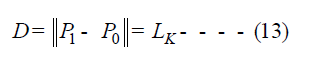The method designed to find the formula for the Distance D, has the following steps,

1. Determination of the 3D Equations of the superior and inferior plane (at an alpha angle with the superior plane).

2. Find the 3D straight line equation (the Pencil Beam quasi-differential cylinder of very small radius) that goes from the collimator output window to the point P0 on the wedge surface.

3. Find the intersection point between the inferior plane of the wedge and this line. That is, the point P1.

4. Calculate the distance D with the above formula.

5. The Distance D mathematical formula is simplified and formatted correctly to carry out convenient approximations (next section Approximations and mathematical analysis).

In Figure 2 we show the basic calculation system of the distance D, and the corresponding Coordinate System. The wedge superior plane, since the main coordinates system is on the center of the superior surface is,

Z = 0 ---------------------------- (14)

To obtain the inferior plane equation we use the vectorial formula for a plane equation in 3D, with a dot product,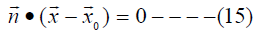That is, the coordinate system is (u1, u2, z), with coordinate origin in the superior plane of the wedge, and Z direction towards the beam propagation (that is, towards the patient body). We use a unitary vector of modulus 1, n, and component u2 null.

Since this vector is perpendicular to the lower wedge plane, it holds an angle alpha with Z Axis, and л/2-α with the u1 axis. The wedge dimensions are 2a length, 2b width, and c depth. Therefore, the maximum depth, d (z value) at the center is d = a tanα; [Figure 3]

The vector group corresponding to the above [see Figure 2] formula is [Eq 16]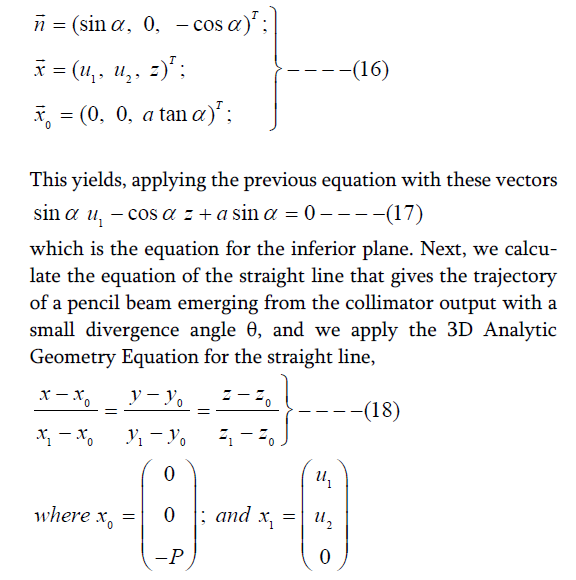We have two points, first, the output point, X0 of the collimator, as detailed above. Second, an arbitrary point at the superior wedge surface, with the coordinate system of the superior wedge surface, that is X1 as detailed above. Therefore, we get the straight line equations,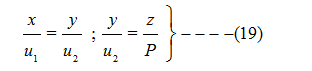And we have the third equation for the inferior plane [Eq 18], so we have a linear system of 3 equations in 3 variables,

sinα u1 - cosα z + a sinα = 0 ;

The solution of the linear equations system is straightforward as follows,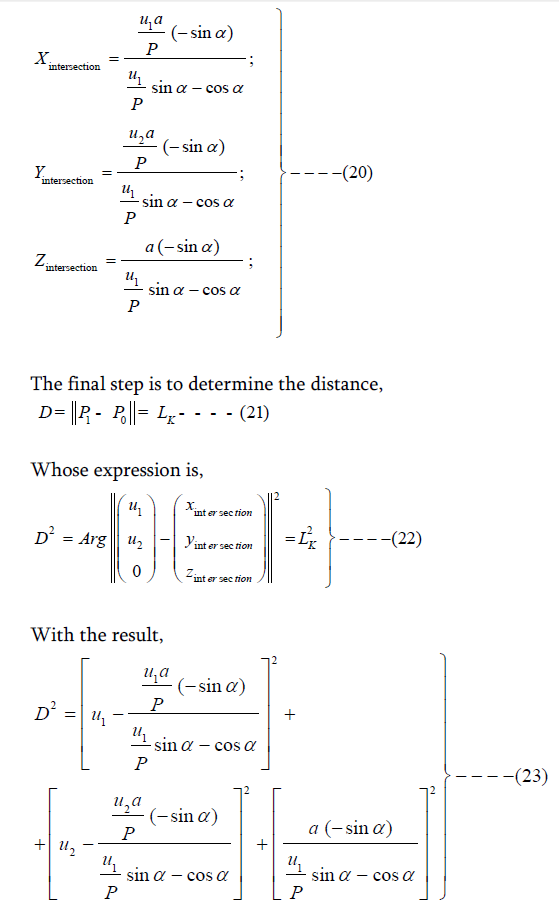According to Figures 2, 3, and 4, we can introduce in the above formula the collimator pencil-beam divergence angle as follows, P = P (θ) cosθ. This substitution works if we want to implement the θ variable in the general formula. In the next section we detail the approximations to make this rather complicated formula easier to calculate and simplify it as much as possible. According to the exponential attenuation factor into the integral dose formula D = LK (Exact Pencil-Beam Pathway Value). This substitution works if we want to implement the θ variable in the general formula. In the next section we detail the approximations to make this rather complicated formula easier to calculate and simplify it as much as possible. According to the exponential attenuation factor into the integral dose formula D = LK (Exact Pencil-Beam Pathway Value).

Approximations and mathematical analysis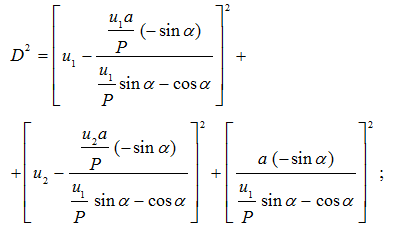Which was set previously as, P = P(θ) cosθ.

Now, we will define two Pencil-Beam Divergence Angles related to θ, namely, θ1  and  θ2  with the trigonometric conditions of Figures 4, and, tan θ1 = u1 / P and tan θ2 = u2 / P.

Proposition 1
In pencil-beam angle decomposition, θ1 and θ2 are always less than or equal to θ.
The objective of this Proposition is to carry out approximations with the smallest angle values possible, making series developments optimal.

Proof
Since (Figure 4),which was the objective to minimize errors in the approximations. Then, we carry out the following changes to include these divergence angle(s) and simplify the initial formula (Exact Pencil-Beam Pathway Formula),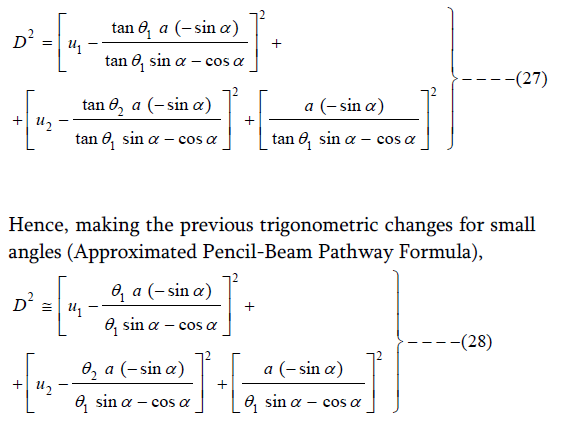Now we have only 5 linear variables within the brackets of the formula, namely, u1, u2, θ1, θ2, θ (optional) and the wedge angles constant α.

Computational simulations and software
A series of simple and basic simulations [Annex A] were carried out to check the accuracy of the approximations and the algebraic development. Simulations were made at the positive quadrant of the thick part of the wedges.The accuracy is given by the error formula of section Error calculations & further approximations. The software used was FreeMat v4.0 (Copyright (c) 2002-2008 by Samit Basu Licensed under the GNU Public License (GPL)) programming and Optimization Subroutines both in single and double precision [C4]. The trajectory of the Pencil Beam for each simulation and the corresponding divergence angle were selected randomly.

The divergence angle interval was chosen narrow, since the divergence angles are usually small in modern accelerators which work with IMRT. It is very important to clarify that when the wedge angle increases, the simulation quadrant for u1 and u2 has to be reduced. The geometrical reason is that there is a Limit Divergence Angle θ for the pencil beam. If this divergence angle is surpassed, the outpoint of the beam after passing through the wedge is located at the lateral side/wall of the wedge (we refer mainly to the broad part of the wide-angle wedge). This inconvenient is especially present when we simulate paths through wide-angle wedges. In Annex A, some details about the Limit Angle are specified, mainly concerning wedges with wide angle, ie, 45º and 60º.

Error calculations & further approximations
The calculation of the Error for a wedge of angle alpha is the Standard RMS Equation where we measure the average quadratic difference between the exact pathway along the wedge material and the approximated path, according to the calculations carried out in section Approximations and mathematical analysis. The RMS Error Formula reads,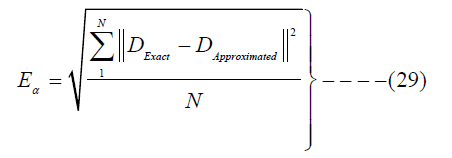Where N is the number of random pathways selected in the simulations. Note that in Annex A Tables, we write positive error values, but negative are also mathematically considered.

The 10 simulations carried out for, i.e. a 30° Wedge give a low RMS Error [Table 2], in the order of 10-3. The tangent approximation in series development results in small errors because the angles at P = 20 cm (distance from the Collimator Output) are also small. The random numbers interval chosen was at the positive quadrant of the broad zone. Usually, the approximated values are larger than the exact ones.

For mathematical analysis, it is both practical and useful to determine what we define as Exponential RMS Error. That is, when we use approximations, the exponential attenuation factor of the wedge filter is set into an interval determined by the numerical errors. Specifically, we are calculating an approximated LK parameter, which is in the exponent of an Integral Exponential Factor (IEF). We have, without approximations,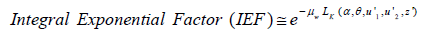Then, since we have determined an approximated LK with RMS Error, the True L’K will be determined within the error interval, so we set a standard Confidence Interval as,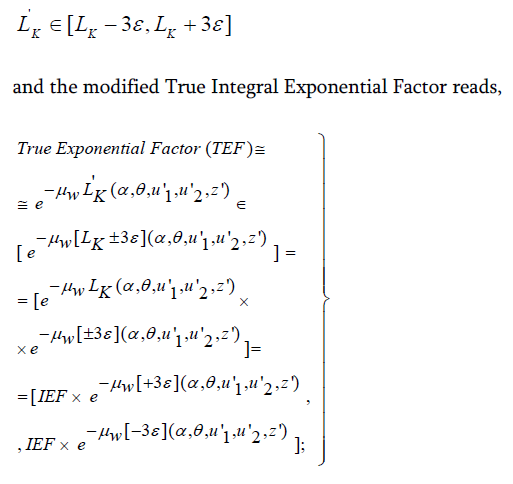In consequence, we define the RMS Error Integral Exponential Factor, to set a trustworthy Confidence Interval (Note that the width of the Confidence Interval can be chosen larger or smaller), as follows,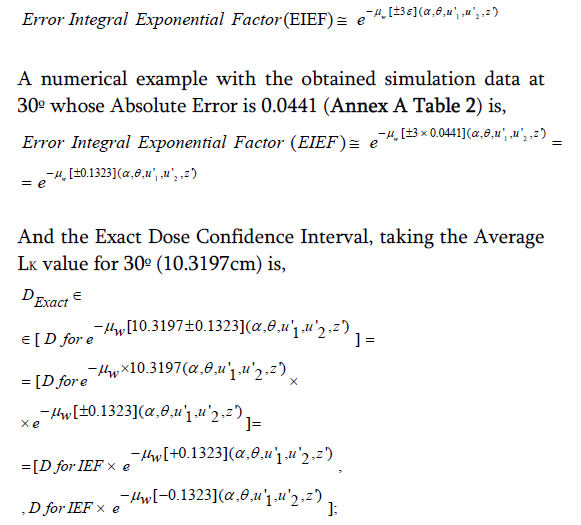With µw values for Alloy tabulated [U2].

We also set the RMS Average Error Integral Exponential Factor, as the average of the distance D Error εA, after we have taken sufficient random RMS measurements along all the wedge surface. These measurements have to take into account the Limit Divergence Angle, θL. Limit Angle mathematical details are explained in section Beam limit divergence angle (LA) concept and formulation with appropriate formulation. Then, this Integral factor reads,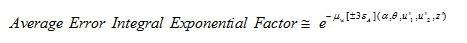The exponential factors always can be approximated by series development. We do not carry out this kind of mathematical framework in this contribution, which will be developed in subsequent publications. The study of how much this kind of approximation of exponential is useful corresponds to approximation theory.

Hence, the Approximated Dose Integral, when using approximation LK (without Confidence Interval), will result,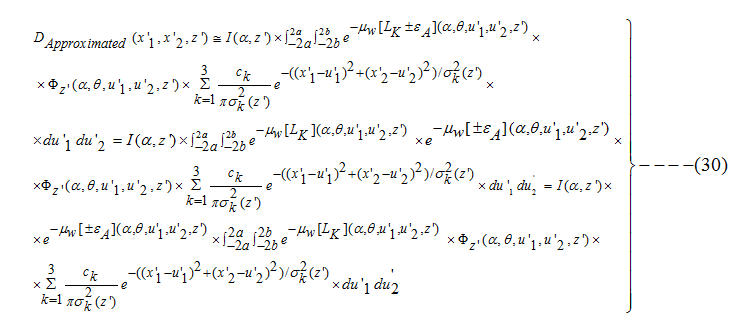And the Average Error Integral Factor has been set outside of the integrand because it is considered average and constant for all the values belonging to the integral Hence, the Approximated Dose Integral, when using approximation LK (without Confidence Interval), will result,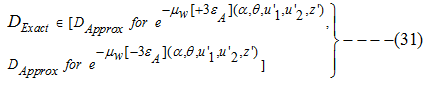A formula which is useful both for industrial manufacturing purposes or treatment planning optimization approximations when using static wedge filters. The Relative RMS Error Formula which has been applied in the tables of Annex A reads,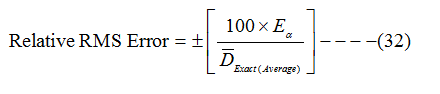where Eα is the RMS Absolute Error [Eq 29]. Note that in Annex A Tables we write positive error values, but negative are also mathematically considered. The Relative Approximation Error is under 5% when the wedge angle is small, 15º or 30º, and rises about 10% when the angle is wider, i.e., 45º, or 60º. The explanation for this increment is that we use only the first term of the tangent series development. Therefore, when both the exact and approximated paths increase, the difference between them becomes higher for trigonometric reasons.  A possible solution is to use more terms of the series, or develop further geometrical approximations.

Beam limit divergence angle (LA) concept and formulation
In previous contributions 16,17, the LA was mathematically defined and developed for wedges. We detail here the main formulas and one sketch of LA, together with a picture of the so-called Conformal Wedge.18 Given a fixed collimator output to wedge surface distance, LA is defined as the maximum angle of divergence that can be reached by the whole radiation beam without emerging at any point of lateral walls of the wedge. Photon-Beam divergence angles values vary around 20 degrees. The Beam minimum divergence depends on the collimator design quality, and in general of the precision engineering manufacturing of the LINACs. LA is useful because of several reasons. Avoids hot spots, sub-optimal dose delivery, planning system software propagation errors, overdose at OARS, and repetition of planning work caused by sub-optimal dose delivery calculations. The LA for a conformal wedge calculation presents some additional difficulties. However, the primary approximation is to take as LA for a Conformal Wedge the value of the deepest step of the wedge. Main formulation for LA in standard wedges is for the principal pencil beamwhere P is the distance between the collimator output and wedge surface (perpendicular, 17) and we have used the constraint for inferior geodesic.17

u12 + u22 = b2

Therefore, to make sure the components of the decomposed beam have a correct output point the following conditions should hold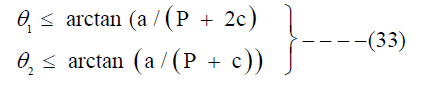Discussion and Conclusion

The pathway in IMRT for static wedges has been determined using an integral Superposition-Convolution Model for Pencil Beam. The radiation delivery model selected was the AAA Superposition-Convolution model with wedge attenuation factor.

The calculation result for 3D is according to the fundamentals of Analytic Geometry. The applied Formulation is the standard in this kind of determinations. We have tried to clarify any kind of complicated mathematical development, formulation and expression. Additional approximations to avoid complicated functions, such as trigonometric or quadratic expressions, have been done taking into account the small divergence angle of the collimator output, θ. These approximations were based mainly on Taylor series development related to trigonometric functions. For instance, the Numerical RMS Error for a 30° Wedge series of 10 simulations is about 10-2 and this magnitude can be considered acceptable, at present investigation stage. We found a Limit Divergence Angle θ related to the central beam, which constitutes an important parameter to avoid the output of the beam mainly through the lateral broad part of the wedge (this phenomenon happens mainly in wedge filters of wide angle). Details about this LA have been explained according to previous publications. Industrial applications of this method are focused on several RT areas. In IMRT, the use of experimentally verified theoretical data of the attenuation of the wedge along its surface, could yield an improved clinical use of the IMRT distribution, and obtain a database for planning system improvements. In addition, it could be employed to develop better attenuation factors for RT mathematical modelling in IMRT or External Beam Radiotherapy (EBRT), according to the geometrical distribution data of the 3D paths along the wedge volume. Furthermore, Nonlinear Optimization Techniques could be used with Multiobjective Techniques 19 for a better combination of the Attenuation Factor(s), wedge zone and IMRT Pencil Beam Intensity Attenuation Factor(s).

Conflict of Interest

The authors declare that they have no conflicts of interest. The authors alone are responsible for the content and writing of the paper.

Received March 13, 2014; Revised May 08, 2014; Accepted May 10, 2014; Published Online May 18, 2014.

Corresponding author: Francisco Casesnoves MSc; Computational Bioengineering Researcher, SIAM (Individual Researcher), Denver, Colorado State, USA.